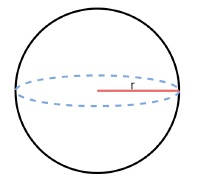# C++ Program to calculate the volume and area of Sphere

The Sphere is a ball-like 3D object whose all sides are curved surfaces. On a sphere, no plane surface is there. The volume of a sphere is actually how much space it is taking in the space. In this article, we shall cover the techniques to calculate the volume and the surface area of a sphere in C++.

A sphere has one basic parameter. The radius 𝑟. We will see the techniques to calculate the volume and area one by one.

## The volume of a Sphere

To calculate the volume of a sphere, we need one input, the radius 𝑟. The formula for the volume is like below −

$$Volume\:=\:\frac{4}{3}\pi\:r^3$$Now let us see the algorithm and implementation for the same.

### Algorithm

• Volume := $\frac{4}{3}\pi\:r^3$.
• Return Volume.

### Example

#include <iostream>
using namespace std;

float solve( float r ) {
float volume;
volume = ( 4 / 3 ) * 3.14159 * ( r * r * r);
return volume;
}
int main() {
cout << "Volume of a sphere with radius r = 2.5 cm, is " << solve( 2.5 ) << " cm^3" << endl;
cout << "Volume of a sphere with radius r = 5in, is " << solve( 5 ) << " in^3" << endl;
}


### Output

Volume of a sphere with radius r = 2.5 cm, is 49.0873 cm^3
Volume of a sphere with radius r = 5in, is 392.699 in^3


## Surface Area of a Sphere

The sphere has only the curved surface, no other plane surface is there. From the radius, we can calculate the surface area by following the simple formula as shown below.

$$Surface\:Area\:=\:4\pi\:r^2$$

The following algorithm is to calculate the surface area for a given Sphere −

### Algorithm

• area := $4\pi\:r^2$.
• Return area.

### Example

#include <iostream>
using namespace std;

float solve( float r ) {
float volume;
volume = 4 * 3.14159 * ( r * r );
return volume;
}

int main() {
cout << "Surface Area of a sphere with radius r = 2.5 cm, is " << solve( 2.5 ) << " cm^3" << endl;
cout << "Surface Area of a sphere with radius r = 5in, is " << solve( 5 ) << " in^3" << endl;
}


### Output

Surface Area of a sphere with radius r = 2.5 cm, is 78.5397 cm^3
Surface Area of a sphere with radius r = 5in, is 314.159 in^3


## Conclusion

The sphere is a ball-like structure in the 3D world. Like other 3D objects, we can calculate the volume and surface area of a sphere. For a sphere, the diameter or the radius is sufficient to express a sphere. From the diameter or radius, we are calculating the area of the curved surface and also the volume of the sphere. The formula for these two is very simple. In the given implementations, the same formula is used in a straightforward manner.